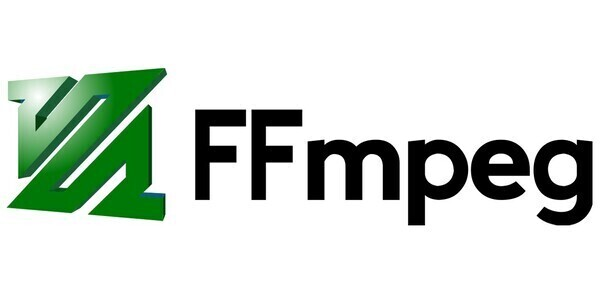close

# How to add an intro to video using ffmpeg

## Learn how to add an intro to video using ffmpeg for batch processingThe command is the following:

`ffmpeg -i "concat:intro_video|original_video" -c copy -bsf:a aac_adtstoasc -max_muxing_queue_size 4096 output_video`

intro_video is the path to the video file that you want as an intro.

original_video is the path to the original video that you want to add the intro to.

Finally, output_video is the path to the new video, that is the original video with the intro added.

What this command does is essentially merge two videos, the first being the intro and the second being the original video. The command executes pretty quickly as no rendering is done. If your intro was already in video format, you can skip everything below.

But what if you want to have an image as a intro instead of a video? Then you will need to transform it into a video first, for that you will use the following command:

`ffmpeg -loop 1 -framerate original_framerate -i intro_image -c:v libx264 -t intro_duration -pix_fmt yuv420p intro_video`
• original_framerate is the framerate of the original video, the "fps". If you don't know it, you can run the following:

`ffmpeg -i original_video`

It will display a lot of information, like in the image below, look for where it says "x fps", x being the number you need.If you're on linux you can use the following hack to get only the framerate:

`ffmpeg -i original_video 2>&1 | sed -n "s/.*, \(.*\) fp.*/\1/p"`
• intro_image is the path to the image you want as an intro.

• intro_duration is the length, in seconds, that you want for the intro.

• intro_video is the result, the video formed by looping intro_image for intro_duration seconds.

Now that you got your image in video format, you can merge it with the original video to add it as an intro.

10/12/2019